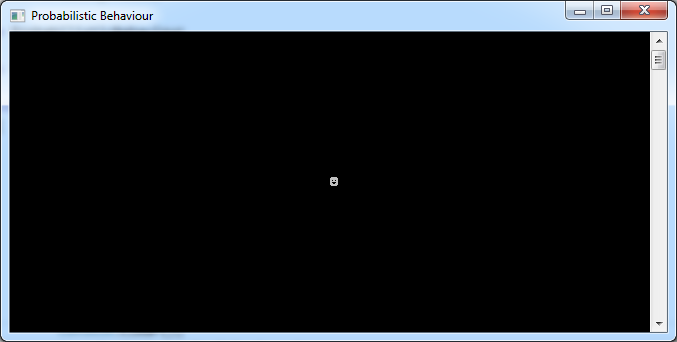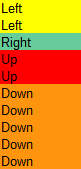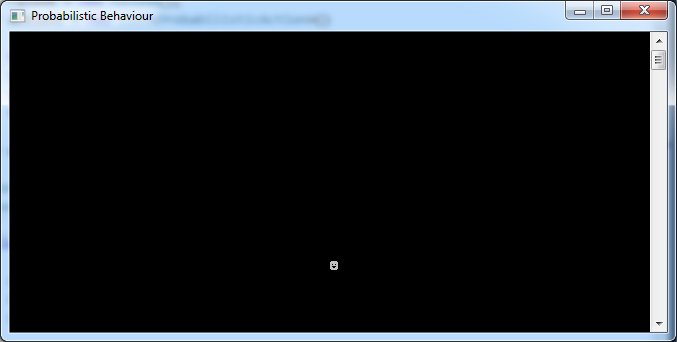# Simulating Probabilistic Behaviour

Let’s say we have a game featuring in-game people (commonly referred to as NPCs). Any game worth its salt will have some form of artificial intelligence (AI) to bring those characters to life to some extent, even if they’re just aimlessly wandering around.

To do this in C# using plain ASCII art, we can start out with the following code, which shows a cutesy ASCII face in the middle of the console window:

```            int x = 40;
int y = 12;
const char person = (char) 2;
const int delay = 1000;

Console.Title = "Probabilistic Behaviour";
Console.CursorVisible = false;

while (true)
{
Console.Clear();
Console.SetCursorPosition(x, y);
Console.Write(person); // show character

// TODO movement code goes here

}
```

This is what you should see after running the code:Now, to make our character wander around randomly is pretty easy. First, declare a `Random` instance near the top of the program:

```            var random = new Random();
```

Then, just replace the “TODO” comment with the following:

```                int direction = random.Next(0, 4); // [0, 3]

switch(direction)
{
case 1: x--; break;
case 2: x++; break;
case 3: y--; break;
case 4: y++; break;
}
```

You should now see the ASCII guy going around randomly. That’s all well and good, but you should realise that this is a uniform distribution: each outcome is just as likely as any other.

Sometimes, that’s not what you want. For example, let’s say you want the following to happen:

1. 20% of the time, the character will go left.
2. 10% of the time, the character will go right.
3. 20% of the time, the character will go up.
4. 50% of the time, the character will go down.

We could represent the above with the following hardcoded logic:

```                double direction = random.NextDouble(); // 0 <= direction < 1

if (direction >= 0 && direction < 0.2)
x--;
else if (direction >= 0.2 && direction < 0.3)
x++;
else if (direction >= 0.3 && direction < 0.5)
y--;
else
y++;
```

That works, and you’ll see the ASCII guy tend to move downwards more than any other direction. But how can we extend this into a generic utility that can accept various different configurations?

It helps if, rather than considering individual probabilities per action, we stack them on top of each other and consider a cumulative probability:

 Action Probability Cumulative Probability Left 0.2 < 0.2 Right 0.1 < 0.3 Up 0.2 < 0.5 Down 0.5 < 1

Stacked on top of each other by probability, the actions would look something like this:In this case we only need to take a random sample between 0 and 1, and see where in the above stack it lands.

To facilitate this, let us first declare a `ProbabilisticAction` class, which represents the mapping between a probability and an action. I’m assuming we don’t need to return anything; if we do, it’s easy to turn this into a generic class.

```    public class ProbabilisticAction
{
public double Probability { get; set; }
public Action Action { get; set; }

public ProbabilisticAction(double probability, Action action)
{
this.Probability = probability;
this.Action = action;
}
}
```

Back in our `Main()`, we can declare a list of such mappings to represent the scenario we had earlier:

```            var actions = new List<ProbabilisticAction>()
{
new ProbabilisticAction(0.2, new Action(() => x--)),
new ProbabilisticAction(0.1, new Action(() => x++)),
new ProbabilisticAction(0.2, new Action(() => y--)),
new ProbabilisticAction(0.5, new Action(() => y++)),
};
```

We then pass these mappings, along with our `Random` instance, into a new class we’ll declare next:

```            var actor = new ProbabilisticActor(actions, random);
```

The `ProbabilisticActor` encapsulates the logic for determining the next action. First, we store the mappings and the `Random` passed in at the constructor:

```    public class ProbabilisticActor
{
private List<ProbabilisticAction> probabilisticActions;
private Random random;

public ProbabilisticActor(List<ProbabilisticAction> probabilisticActions,
Random random)
{
this.probabilisticActions = probabilisticActions;
this.random = random;
}
}
```

To avoid confusion, we also want to ensure that the probabilities passed in actually add up to 1:

```        public ProbabilisticActor(List<ProbabilisticAction> probabilisticActions,
Random random)
{
if (probabilisticActions == null)
throw new ArgumentNullException(nameof(probabilisticActions));
if (probabilisticActions.Select(mapping => mapping.Probability).Sum() != 1.0)
throw new ArgumentException("Probabilities must add up to 1!");

this.probabilisticActions = probabilisticActions;
this.random = random;
}
```

Now, we can actually add the logic that picks the next action to execute. This is done by taking a random sample and seeing where it falls in the probability space:

```        public void DoNextAction()
{
double sample = random.NextDouble();

foreach(var mapping in probabilisticActions)
{
double probability = mapping.Probability;
sample -= probability;

if (sample <= 0)
{
var action = mapping.Action;
action();
break;
}
}
}
```

All we have left is to replace the logic in `Main()` with a call to this new method:

```            while (true)
{
Console.Clear();
Console.SetCursorPosition(x, y);
Console.Write(person); // show character

actor.DoNextAction();